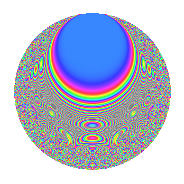# Properties

 Label 525.4.rLevel $525$ Weight $4$ Character orbit 525.r Rep. character $\chi_{525}(424,\cdot)$ Character field $\Q(\zeta_{6})$ Dimension $144$ Sturm bound $320$

# Related objects

## Defining parameters

 Level: $$N$$ $$=$$ $$525 = 3 \cdot 5^{2} \cdot 7$$ Weight: $$k$$ $$=$$ $$4$$ Character orbit: $$[\chi]$$ $$=$$ 525.r (of order $$6$$ and degree $$2$$) Character conductor: $$\operatorname{cond}(\chi)$$ $$=$$ $$35$$ Character field: $$\Q(\zeta_{6})$$ Sturm bound: $$320$$

## Dimensions

The following table gives the dimensions of various subspaces of $$M_{4}(525, [\chi])$$.

Total New Old
Modular forms 504 144 360
Cusp forms 456 144 312
Eisenstein series 48 0 48

## Trace form

 $$144q + 288q^{4} - 48q^{6} + 648q^{9} + O(q^{10})$$ $$144q + 288q^{4} - 48q^{6} + 648q^{9} + 112q^{11} - 300q^{14} - 1040q^{16} - 132q^{19} + 24q^{21} - 288q^{24} + 432q^{26} + 512q^{29} + 1124q^{31} - 704q^{34} + 5184q^{36} - 84q^{39} - 1648q^{41} - 852q^{44} - 1192q^{46} - 988q^{49} + 336q^{51} - 216q^{54} - 5604q^{56} - 3728q^{59} + 2144q^{61} + 928q^{64} + 2424q^{66} + 2016q^{69} + 1792q^{71} + 5936q^{74} + 2152q^{76} + 2612q^{79} - 5832q^{81} - 3672q^{84} - 4260q^{86} - 6544q^{89} + 3340q^{91} - 3080q^{94} + 5268q^{96} + 2016q^{99} + O(q^{100})$$

## Decomposition of $$S_{4}^{\mathrm{new}}(525, [\chi])$$ into newform subspaces

The newforms in this space have not yet been added to the LMFDB.

## Decomposition of $$S_{4}^{\mathrm{old}}(525, [\chi])$$ into lower level spaces

$$S_{4}^{\mathrm{old}}(525, [\chi]) \cong$$ $$S_{4}^{\mathrm{new}}(35, [\chi])$$$$^{\oplus 4}$$$$\oplus$$$$S_{4}^{\mathrm{new}}(105, [\chi])$$$$^{\oplus 2}$$$$\oplus$$$$S_{4}^{\mathrm{new}}(175, [\chi])$$$$^{\oplus 2}$$

## Hecke characteristic polynomials

There are no characteristic polynomials of Hecke operators in the database